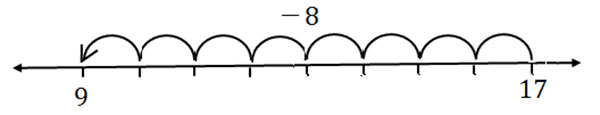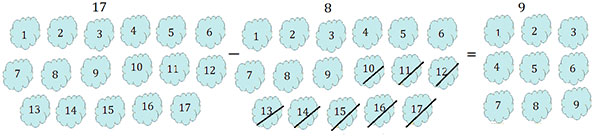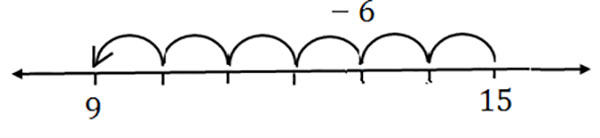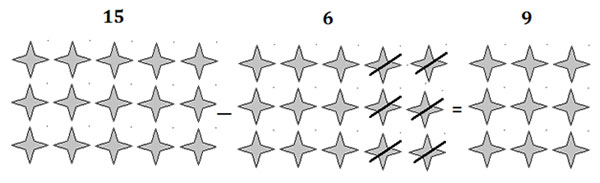# Subtracting a Number From a 2-digit Number

Subtract the numbers 17 − 8

### Solution

Method 1

We subtract the numbers using a number line here. On the number line, start from the larger number 17 and then make 8 jumps to the left to land on 9. This is the difference between 17 and 8.Method 2

Counting backwards

We represent number 17 with 17 little diamonds. Since 8 is to be subtracted from 17, we strike off 8 diamonds while counting backward. The 9 diamonds that are left, are the result of the subtraction.

17 – 8 = 9Subtract the numbers 15 − 6

### Solution

Method 1

We subtract the numbers using a number line here. On the number line, start from the larger number 15 and then make 6 jumps to the left to land on 9. This is the difference between 15 and 6.Method 2

Counting backwards

We represent number 15 with 15 little stars. Since 6 is to be subtracted from 15, we strike off 6 stars while counting backward. The 9 stars that are left, are the result of the subtraction.

15 – 6 = 9